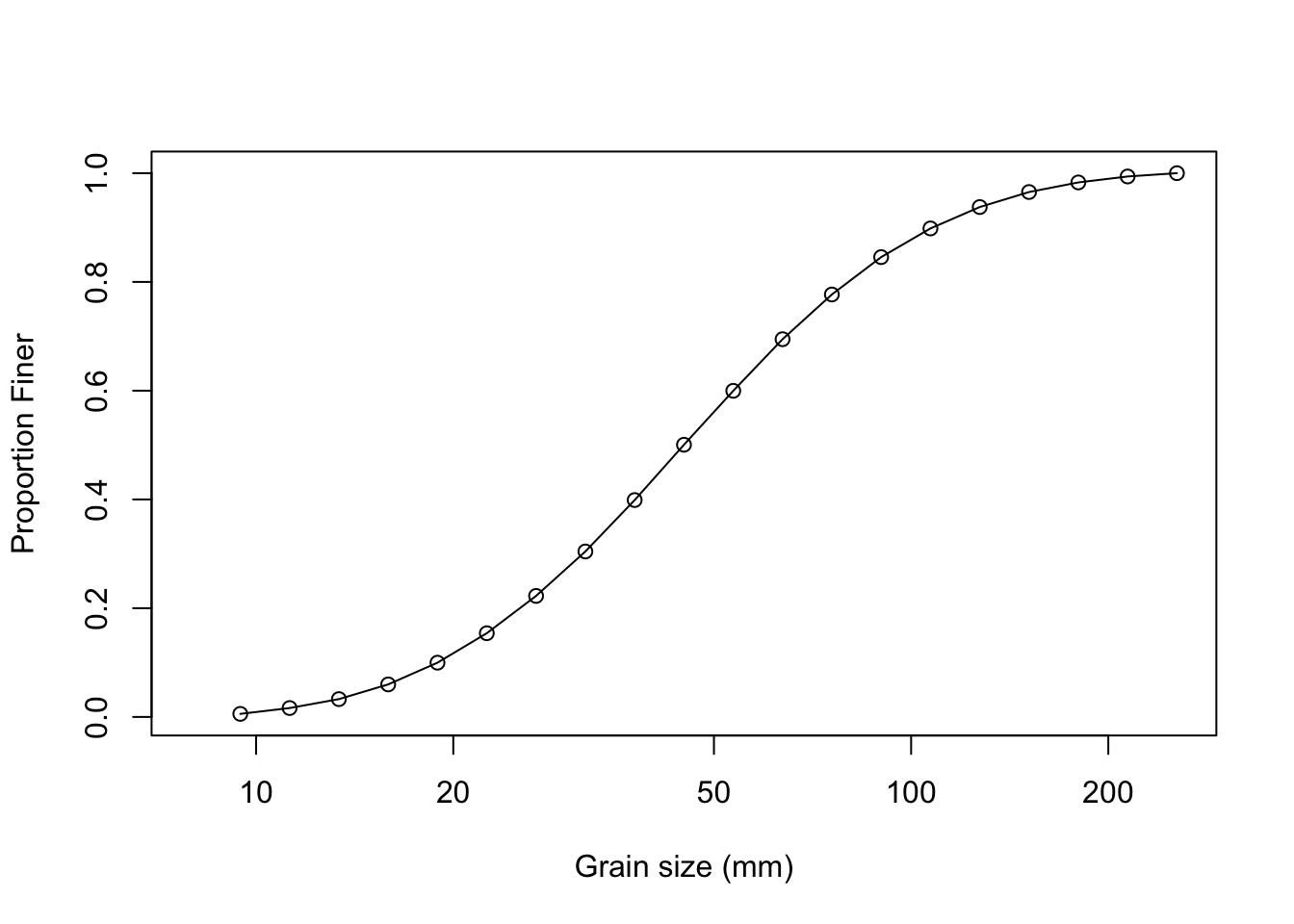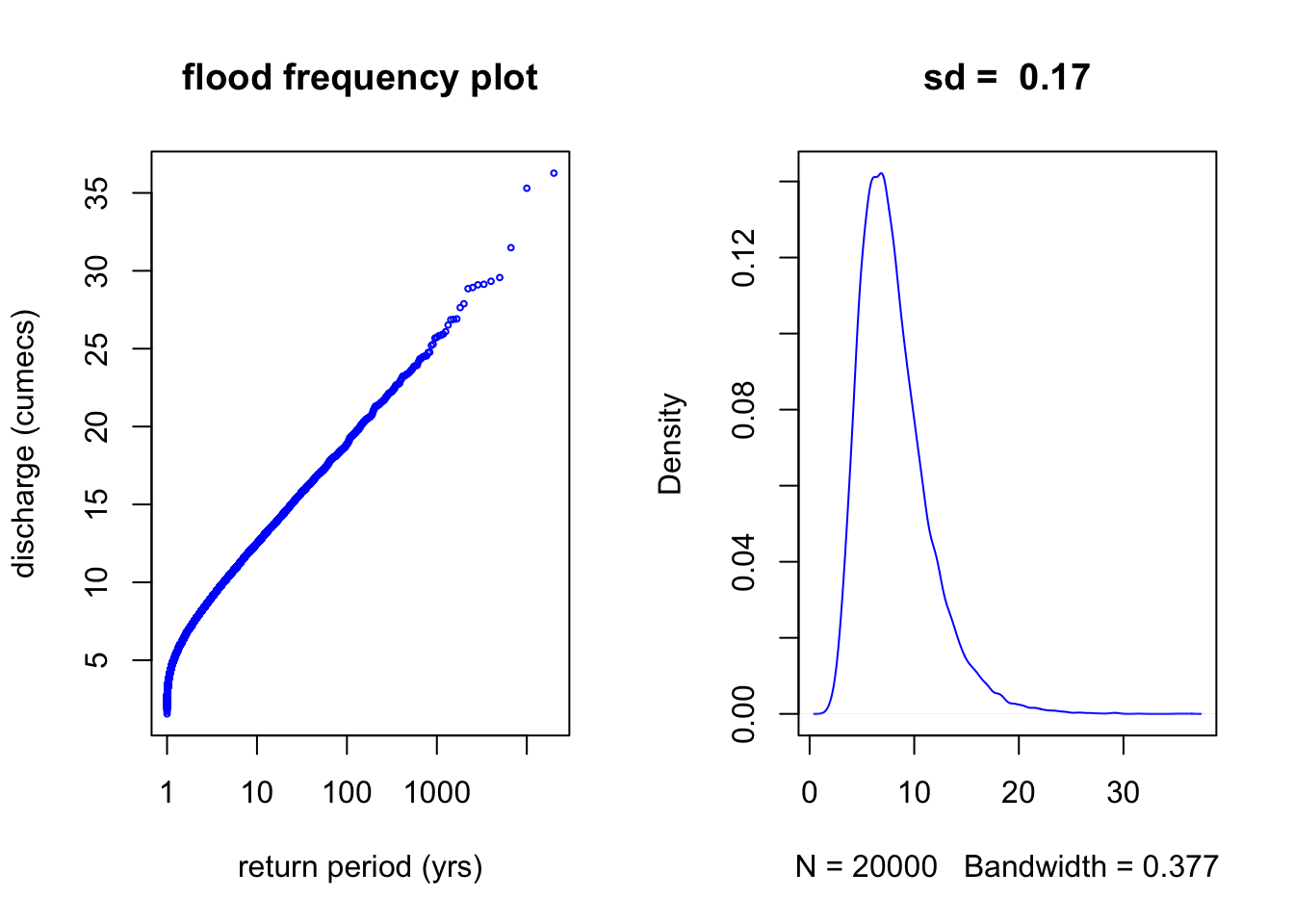# stochasim

STOCHASIM R Package

# Introduction

Based on the PhD work done by Sarah Davidson at UBC, we have created a R package that implements the STOCHASIM model for channel migration. The model investigates how the frequency and magnitude of bank erosion changes with flood frequency distribution, bed surface grain size distribution, and channel slope. The package includes functions to easily simulate the bed surface grain size distribution, and the flood frequency distribution, as well as functions for a series of analyses of the model results. This post presents instructions for installing the package, generating the inputs for the model, running the model, and analysing the model results.

# Installation

The package is currently available on GITHUB, but you will need to install the `devtools` package to access it.

``````#install.packages("devtools")  #if you have not done so already, install devtools
install_github("bceaton/stochasim")  #install stochasim from github``````
``````## Skipping install of 'stochasim' from a github remote, the SHA1 (0c65ac24) has not changed since last install.
##   Use `force = TRUE` to force installation``````
``library(stochasim)  #load the stochasim package``

# Preparing the data inputs

For this example, we consider the dynamics of a steep gravel bed stream. The reach gradient is 0.017 m/m. This is a constant used to run the model.

## grain size data

For our example, let’s assume we know that the surface \(D_{50} \approx 45\) mm, and the largest sediment on the channel bed is about 200 mm. Rather than trying to input a specific grain size distribution, we will simulate it using the `sim_gsd` function, and specifying the `D50` and a variable `sp` that controls the spread of a log-normal distribution. The code to generate the required data frame (`gsd`) is shown below, along with code to estimate the \(D_{50}\), \(D_{84}\), and \(D_{99}\) (stored in the variable `sizes`). We adjust the value of `sp` to match our knowledge of the surface GSD.

``gsd = sim_gsd(D50 = 45, sp = 1, Map = T)````````sizes = approx(x = gsd\$cdf, y = gsd\$size_class, xout = c(0.5, 0.84, 0.99))
print(round(sizes\$y))``````
``##   45  89 202``

## flood frequency data

For our example, let us assume that the mean annual flood is about 7.4 m\(^3\)/s, and the largest flood in 30-year record is about 15 m\(^3\)/s. We can also estimate the flood frequency distribution using a log-normal function by specifying the mean of the distribution and the maximum flood we expect to occur in the function `sim_flood`. We also specify the number of discharge values to extract from the distribution, which determines the number of years that the `stochasim` model will run.

``````mean.discharge = 7.5
max.discharge = 25
n.years = 20000
Qvals = sim_flood(Qmean = mean.discharge,
Qmax = max.discharge,
n = n.years,
Map = T)``````# Running the model

The simulation is run by simply calling the `run_stochasim` function, and storing the output in the dataframe `results`.

``````results = run_stochasim(gsd,
Qvals,
slope = 0.017)``````
``##  "proportion of the bed that is stable at threshold stress is:  0.06"``

That’s all there is to running the model. It may take a while, depending on the number of years being simulated, but otherwise it is straightforward.

# Visualizing the model results

We have created a function that plots the time series for discharge and the simulated channel width for years 500 to 1000 of the simulation, so that the modeler can get a sense of what year-to-year variations look like.

``plot_timeseries(results)``